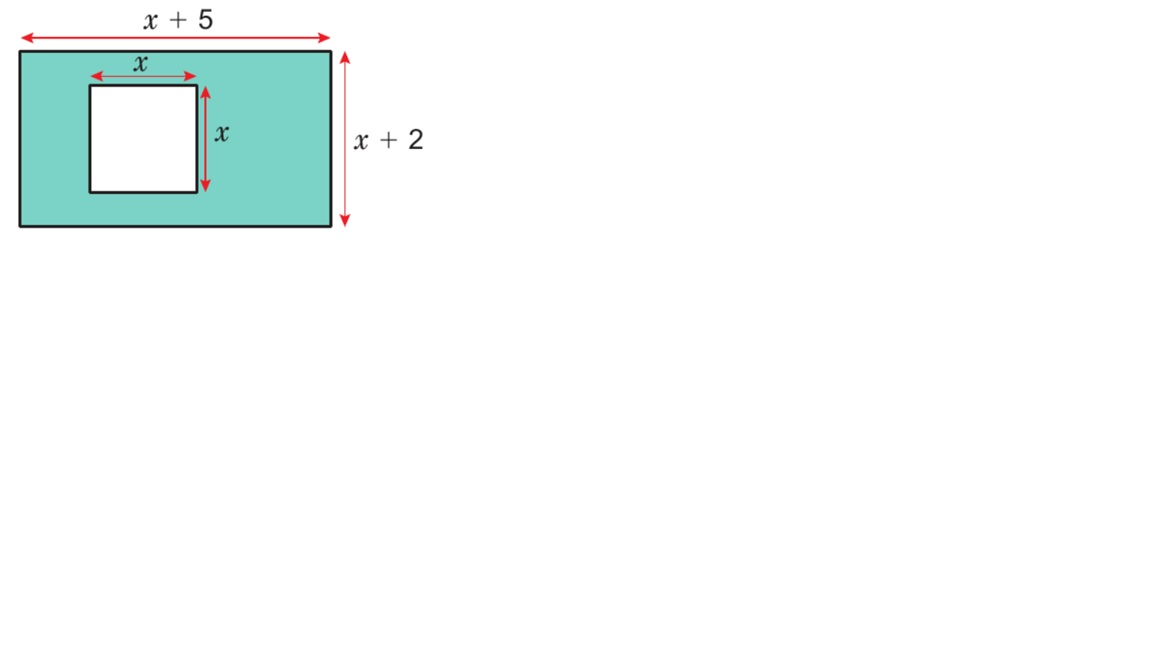## 11 Algebra-expression for the area of the rectangle

###### The problem

A rectangular piece of paper has length  (x +5) cm and width ( x +2 ) cm.

A square with sides of length, x cm is removed.

a) Write an expression for the area of the rectangle before the square is cut out. Expand the brackets.

b) Write an expression for the shaded area

c) Find x if the shaded area is 31$c{m}^{2}$.

###### The solution

a) Expression for the area of the rectangle before the square is cut.

( x + 5) ( x + 2)Ruhi Quadri Published on 06 Nov 2020